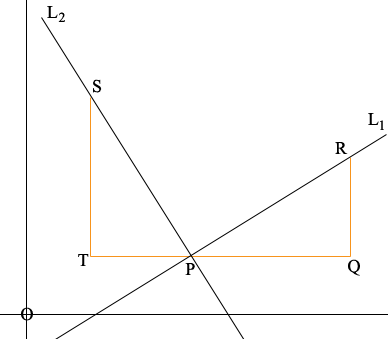SEARCH HOMEMath Central Quandaries & QueriesQuestion from Alister, a student: how do i prove that the product of gradients between 2 perpendiculars lines equal to -1....Hi Alister,

I can draw a diagram for you which hopefully will point you in the right direction.L1 and L2 are perpendicular and P is their point of intersection. R is a point on L1 different from P and Q is such that PQ is horizontal and QR is vertical. Extend QP to T so that |TP| = |QR|. The vertical line through T meets L2 at S.

What is the slope of L1?
What is the slope of L2?
What can you say about the measures of the angles QRP and RPQ?
What can you say about the triangles PQR and PST?

PennyMath Central is supported by the University of Regina and The Pacific Institute for the Mathematical Sciences.